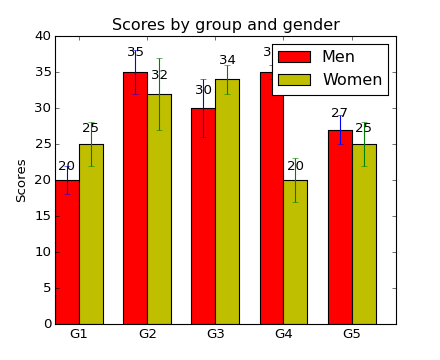### We're updating the default styles for Matplotlib 2.0

Learn what to expect in the new updates#### Previous topic

api example code: agg_oo.py

#### Next topic

api example code: bbox_intersect.py

# api example code: barchart_demo.py¶```#!/usr/bin/env python
# a bar plot with errorbars
import numpy as np
import matplotlib.pyplot as plt

N = 5
menMeans = (20, 35, 30, 35, 27)
menStd =   (2, 3, 4, 1, 2)

ind = np.arange(N)  # the x locations for the groups
width = 0.35       # the width of the bars

fig, ax = plt.subplots()
rects1 = ax.bar(ind, menMeans, width, color='r', yerr=menStd)

womenMeans = (25, 32, 34, 20, 25)
womenStd =   (3, 5, 2, 3, 3)
rects2 = ax.bar(ind+width, womenMeans, width, color='y', yerr=womenStd)

# add some text for labels, title and axes ticks
ax.set_ylabel('Scores')
ax.set_title('Scores by group and gender')
ax.set_xticks(ind+width)
ax.set_xticklabels( ('G1', 'G2', 'G3', 'G4', 'G5') )

ax.legend( (rects1, rects2), ('Men', 'Women') )

def autolabel(rects):
# attach some text labels
for rect in rects:
height = rect.get_height()
ax.text(rect.get_x()+rect.get_width()/2., 1.05*height, '%d'%int(height),
ha='center', va='bottom')

autolabel(rects1)
autolabel(rects2)

plt.show()
```

Keywords: python, matplotlib, pylab, example, codex (see Search examples)